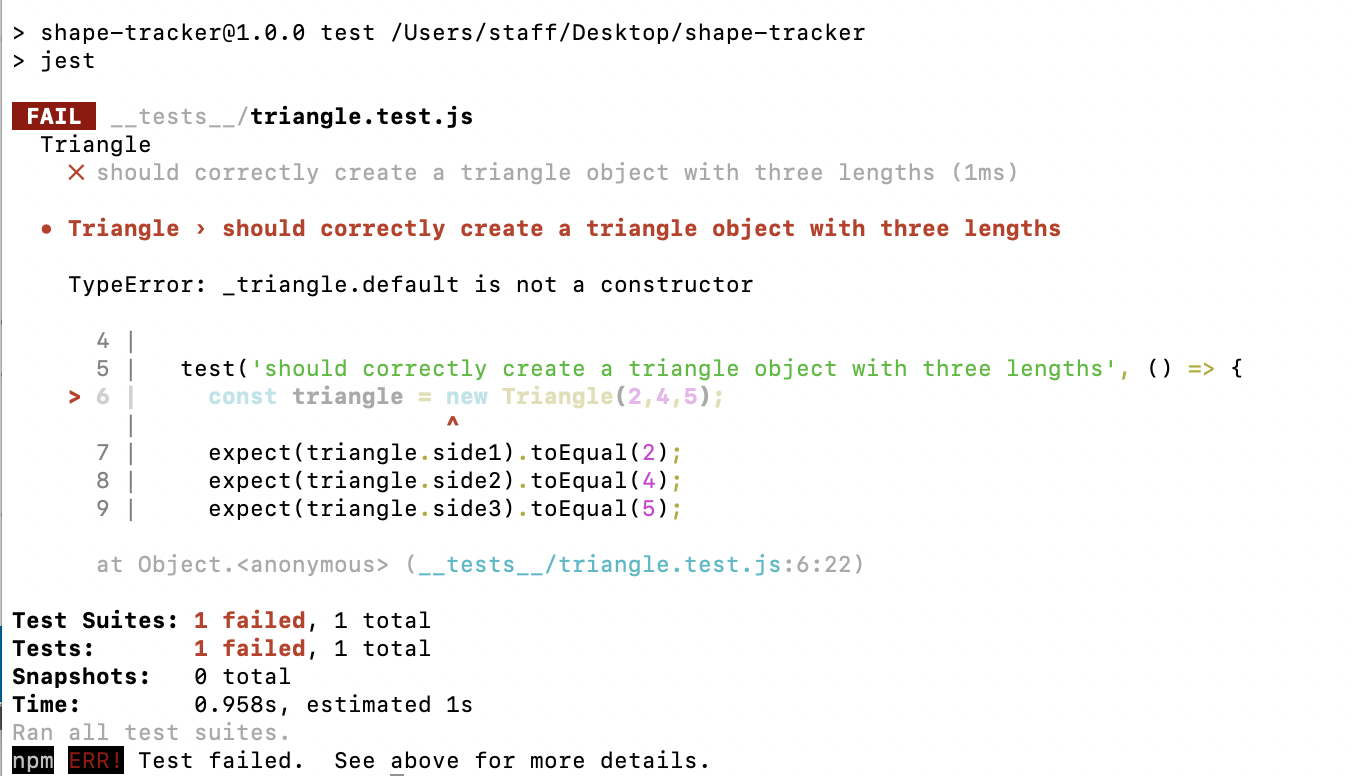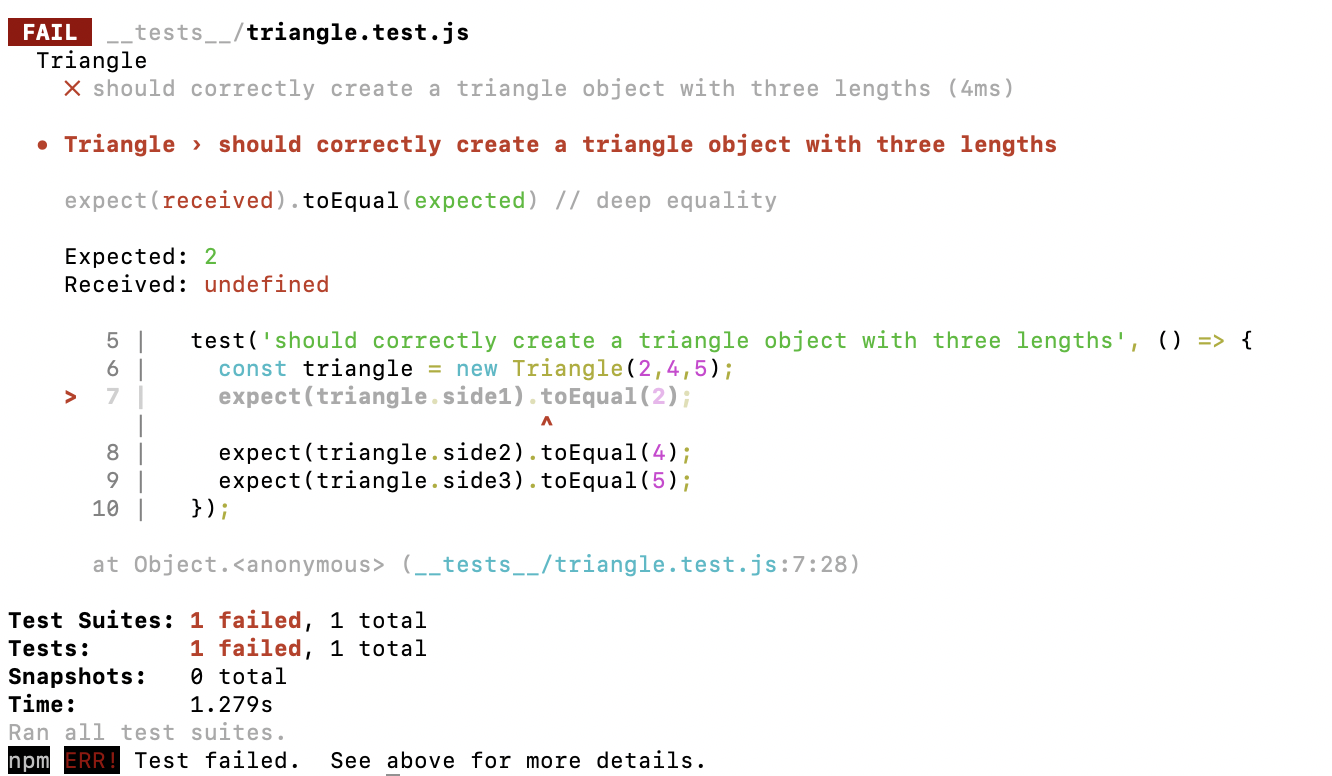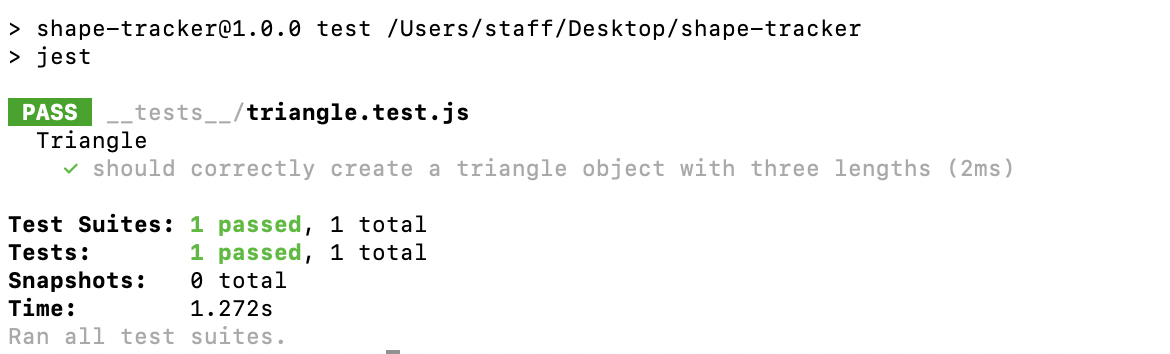Lesson Sunday

Now that Jest is set up, it's time to test some code. We're now ready to write the business logic to check if three lengths make a triangle (and what kind of triangle they make) in our shape tracker application. As we write our business logic, we'll use the Red, Green, Refactor workflow, and commit our code after each passing test.

In order to effectively demonstrate the Red, Green, Refactor workflow, we'll need to start from scratch, which means we'll need to delete all of our business logic in `triangle.js`. Go ahead and do so now.

Also note that over the last few course sections, we've been adding pseudocode tests to our READMEs. We've done so with specific goals in mind: to learn how to use a TDD approach as a problem-solving tool and also to prepare for writing tests using Jest. Now that we're actually writing tests with Jest, it's no longer necessary to add pseudocode tests in your READMEs. Instead, we'll shift our focus to comprehensively testing our code with Jest.

## Review: Writing Tests before Logic

Students often describe that it's an awkward process to write a test before the actual logic has been written that the test is supposed to test for. This is certainly true, and can take some getting used to.

One thing that's helpful to remember is that we're shaping the name and functionality of a function when we write the test. We decide what our function is called, how we call it (if there's any arguments, or not), and what it returns all before actually coding it. And this is exactly the point of this Test-Driven Development — to decide where we're going before we start coding — and to do so incrementally.

## Test 1: Should Correctly Create a Triangle Object with Three Lengths

With the business logic erased in `src/triangle.js`, we're ready to recreate the business logic for our `Triangle` object using TDD.

Let's write our first test. A lot of the content will look familiar because we've written pseudocode tests that look very similar to Jest tests.

__tests__/triangle.test.js
``````import Triangle from './../src/triangle.js';

describe('Triangle', () => {

test('should correctly create a triangle object with three lengths', () => {
const triangle = new Triangle(2,4,5);
expect(triangle.side1).toEqual(2);
expect(triangle.side2).toEqual(4);
expect(triangle.side3).toEqual(5);
});
});
``````

The test above simply checks to see if the constructor works and properly instantiates a `Triangle` object with three properties: `side1`, `side2`, and `side3`. Remember that we should always start by testing the smallest possible behavior. In this case, it makes sense to check if we can properly instantiate a `Triangle` object.

Let's go over the different parts of this test including the new functions and methods that we're using from Jest to run our tests:

• The `describe()` function.
• The `test()` function.
• The `expect()` function.
• And 'matcher' methods, like `toEqual()`.

### 1. First, we must always import any necessary code from other files.

``````import Triangle from './../src/triangle.js';
``````

In this case, we need to `import Triangle from './../src/triangle.js';`. That way, Jest can actually access the file that needs to be tested. And don't forget that we used Babel to make sure Jest understands import and export statements — this is exactly the issue that Babel solves for us.

### 2. Then we set up a `describe()` statement.

``````describe('Triangle', () => {
// tests go in here
});
``````

We've been using describe to group our pseudocode tests so far so this term should be very familiar now. However, in the context of automated testing, we're going to be more specific about what it means: we use `describe()` to define a suite. A suite allows us to group and organize tests. There's a couple things to note here:

• We don't need to describe this suite as a `Triangle`. We could describe it as `'the Triangle and all its prototypes'`. The description is there to make our code more readable.
• Our suite should be described in a concise and descriptive way. Since it's a suite that describes `Triangle` and its prototypes, describing it as a `Triangle` makes sense. If we were to test other shapes such as squares and circles, they'd have their own `describe` block.
• We also have some new JS syntax called arrow notation: `() =>`. When we use this syntax, it's the same thing as saying `function()`, though, there are some other benefits to using arrow notation that function declarations do not have. We will cover arrow notation further in a future lesson. You can use either `() =>` or `function()` in your Jest tests. Keep in mind that the Jest documentation uses `() =>`.

### 3. Next, within our `describe()` block, we create a test that calls on the function we are testing.

``````test('should correctly create a triangle object with three lengths', () => {
const triangle = new Triangle(2,4,5);
expect(triangle.side1).toEqual(2);
expect(triangle.side2).toEqual(4);
expect(triangle.side3).toEqual(5);
});
``````

The term test should be very familiar at this point! Jest tests begin with `test()` — very similar to the pseudocode we've been using. Once again, we describe the content of the test, which generally begins with the word "should." Jest doesn't care what we put in this string. It's there so we can better communicate our intentions as developers. As always, we should try to be specific without being overly verbose.

Within the test, we can run any JavaScript code we need. Because this test is testing whether we can properly create an instance of a Triangle, we just need to call the `Triangle()` constructor to instantiate a `triangle`:

``````const triangle = new Triangle(2,4,5);
``````

### 4. Finally, every test includes one or more expectation statements that use a matcher method.

``````expect(triangle.side1).toEqual(2);
expect(triangle.side2).toEqual(4);
expect(triangle.side3).toEqual(5);
``````

We have 3 expectation statements, which are each made up of calling the `expect()` function and a matcher method like `toEqual()`. In these statements we describe what we expect our result to be, as compared to the actual result from calling on our business logic function.

Expectation statements are common across test frameworks. It's also common to call an expectation an assertion, and these terms can be used interchangeably.

We pass in the value that we've derived from running the `Triangle()` constructor in the `expect()` function, and then in the `toEqual()` method we pass in a hardcoded value that represents our expected result from calling the `Triangle()` constructor. If we wanted to describe this in pseudocode, here's what this looks like:

``````// the arguments
``````

The `toEqual()` method is called a matcher. A matcher determines how `derivedValueFromBusinessLogic` should match `resultWeExpect`. Or, how the actual result should match our expected result. In the test that we wrote, if the results are equal, the test returns true and the test will pass. If the results aren't equal, the test returns false and the test will fail.

In the pseudocode tests we've written up until now, we always listed what we expected our output to equal as if we were using Jest's `toEqual()` method. Well, Jest has many other matchers we can use. For instance, we can check if a value is less than another value, if it's true or false, and so on. For more information on matchers, see the Jest documentation on using matchers.

### A Best Practice: A Test Should Test for One Thing, No Matter How Many Expectation Statements There Are

As stated previously, the test we wrote has 3 expectation statements:

``````test('should correctly create a triangle object with three lengths', () => {
const triangle = new Triangle(2,4,5);
expect(triangle.side1).toEqual(2);
expect(triangle.side2).toEqual(4);
expect(triangle.side3).toEqual(5);
});
``````

Often tests will have just one expectation statement, unlike in the test above. However, you can really have as many expectations as you need, as long as your test is testing for just one thing. In this case, all of the expectations are testing the same thing: that the `Triangle()` constructor can correctly create a `Triangle` object with the properties it needs.

Now that we have a test written for our `Triangle()` constructor, and no logic in `triangle.js`, we're ready to complete the red phase or Red, Green, Refactor to verify that our tests fails. In the root of the Shape Tracker directory, run the npm test script:

``````\$ npm test
``````Jest provides nice color coding for us. Green means the test passed while red indicates a fail. We've got a pretty obvious fail here, but it's not a meaningful one.

Remember how we said there are good and bad fails? Well, in this case, the test throws a `TypeError: _triangle.Triangle is not a constructor` error when we try to instantiate a triangle.

Why is it throwing the error? Well, we don't have a constructor now. Our goal is to test the constructor itself, not the absence of a constructor. If a test can't find a method, file, or constructor and fails as a result, that is always going to be a bad fail. It's not really testing anything — it's just demonstrating that our code isn't wired up properly.

Another way to tell this is a bad fail is because our expectation is never reached. The error happens immediately so our test doesn't run successfully.

## A Good Fail

Let's update our code to add our constructor back. We won't add any properties yet.

src/triangle.js
``````export default function Triangle(side1, side2, side3) {

}
``````

We now have a constructor that's successfully exported, even if no properties are initialized when the constructor makes a new instance of a `Triangle` object. If we run `\$ npm test` again, our test will fail — as expected — but in a different way:The failure message highlights the values of the expected value versus the received (actual or derived) value:

``````expect(received).toEqual(expected) // deep equality

Expected: 2
``````

The key difference here is that we've actually reached our expectations. This way, we know that our test is properly connected to our code — and that we have a basic constructor in place. We expect our test to fail because our constructor doesn't have any properties yet.

It's important to get our tests to fail first because otherwise we might get a false positive. This could happen if we wrote the test incorrectly or if our tests are testing something other than we intended.

The red part of the RGR workflow is now complete, at least for our first test. That means we are ready to move onto getting the test passing — making it green.

## Passing Our First Test

Well, this part is simple. We just need to add our constructor's properties:

src/triangle.js
``````export default function Triangle(side1, side2, side3) {
this.side1 = side1;
this.side2 = side2;
this.side3 = side3;
}
``````

Once we do that, we can run `\$ npm test` again.As we can see, our test is color coded green now. We've passed our first test — and we can see why this is called the red-green-refactor workflow.

There are no opportunities to refactor — at least not yet — but don't forget that we should always look for opportunities to improve our code after each passing test.

Now that we have a passing test, it's important to commit your code. You should always commit your code after each passing test. Think of it as being like a save point where all is well and everything is working correctly — you can always return to this save point later if your code goes south.

Also, in the real world, we'll always want to commit after each passing test anyway to document our work. Just to clarify, you should commit not only the updated source code but also the updated tests.

## How to Test Functions that Have Multiple Behaviors

There's one other important thing to note before we move on. Only one test is listed as passing even though we have three expectations. Is this an issue? Sometimes, but not always. Your unit test should always be testing one unit of code, meaning a single behavior. In this test, we're testing that the constructor can create a `Triangle` object with 3 sides — this is just one behavior we're testing for, the creation of a triangle. In this case, it's fine to have multiple expectation statements in one test.

However, what if our constructor handles creating a `Triangle` object with 3 sides and calculated whether or not the Triangle is in fact a triangle? Consider the code snippet below that borrows code from the `Triangle.prototype.checkType()` method:

``````export default function Triangle(side1, side2, side3, height) {
this.side1 = side1;
this.side2 = side2;
this.side3 = side3;
this.isTriangle = ((this.side1 > (this.side2 + this.side3)) || (this.side2 > (this.side1 + this.side3)) || (this.side3 > (this.side1 + this.side2))) ? "not a triangle" : "this is a triangle";
}
``````

In this example, we're using a ternary operator, which takes this format:

``````condition ? outcome if condition is truthy : outcome if condition is falsey`
``````

There are 3 sections in the ternary, separated by a question mark `?` and a colon `:`. In the first section, we state the condition that should be evaluated. In the next section after the `?`, we have the expression that will execute if the condition is truthy. Then in the last section after the `:`, we have the expression that will execute if the condition is false.

In our example, `this.isTriangle` will be set to the value of `"not a triangle"` or `"this is a triangle"` depending on whether this condition:

``````((this.side1 > (this.side2 + this.side3)) || (this.side2 > (this.side1 + this.side3)) || (this.side3 > (this.side1 + this.side2)))
``````

returns as true or false.

Now, does the Triangle constructor include one behavior or two behaviors? Would we write a test for creating a `Triangle` object with 3 sides and an isTriangle property? Or would we write two tests, one for determining whether the sides make a true triangle, and the other for creating a triangle object with 3 sides?

There's no right answer. However, we want you to ask these questions so that you are considering how to write your tests so that they clearly communicate the different behaviors of your application, no matter how it is structured in the code.

Sometimes development teams or companies have specific guidelines for testing that could answer the question of whether to write two tests, or another expectation, however this is certainly not always the case.

Now, let's consider this code:

``````export default function Triangle(side1, side2, side3, height) {
this.side1 = side1;
this.side2 = side2;
this.side3 = side3;
this.isTriangle = ((this.side1 > (this.side2 + this.side3)) || (this.side2 > (this.side1 + this.side3)) || (this.side3 > (this.side1 + this.side2))) ? "not a triangle" : "this is a triangle";
this.isScalene = ((this.side1 !== this.side2) && ((this.side1 !== this.side3)) && ((this.side2 !== this.side3))) ? "is scalene" : "not scalene";
this.isEquilateral = ((this.side1 === this.side2) && (this.side1 === this.side3)) ? "is equilateral" : "not equilateral";
}
``````

This constructor is starting to get code smell! The ternary operator is hard to read, and the constructor is doing more than just creating a `Triangle` object. In fact, this is why we have a `Triangle.prototype.checkType()` method to determine what type of triangle we have!

Those concerns aside, how many tests would you write for this constructor?

If we had one test for the constructor with multiple expect statements covering all of the behavior, we'd be testing the `Triangle` constructor, but it wouldn't be obvious that means we're:

1. Creating a `Triangle` object with 3 sides, and
2. Determining if the inputted sides actually make a triangle, and,
3. Determining if the triangle is scalene, and
4. Determining if the triangle is equilateral.

When we distinguish each behavior, it becomes clear that we are doing much more than just creating a `Triangle` object, and that it's likely better to have multiple tests that describe and test each behavior.

In the end, it's OK to have multiple expectations in a single test, but make sure that each test is covering just one behavior.

Next up, let's continue applying TDD and the RGR workflow to the `Triangle.prototype.checkType()` method.